# RD Sharma Solutions for Class 10 Maths Chapter 1 Real Numbers Exercise 1.2

This exercise helps students understand an important property of integer called Euclid’s Division Algorithm. Further, that property is applied to find the HCF of two or even three numbers. If you feel any difficulty in understanding any problem the RD Sharma Solutions Class 10 is the right resource available for your need. For a detailed solution to these exercise problems students can download RD Sharma Solutions for Class 10 Maths Chapter 1 Real Numbers Exercise 1.2 PDF, which is provided below.

## RD Sharma Solutions for Class 10 Maths Chapter 1 Real Numbers Exercise 1.2 Download PDF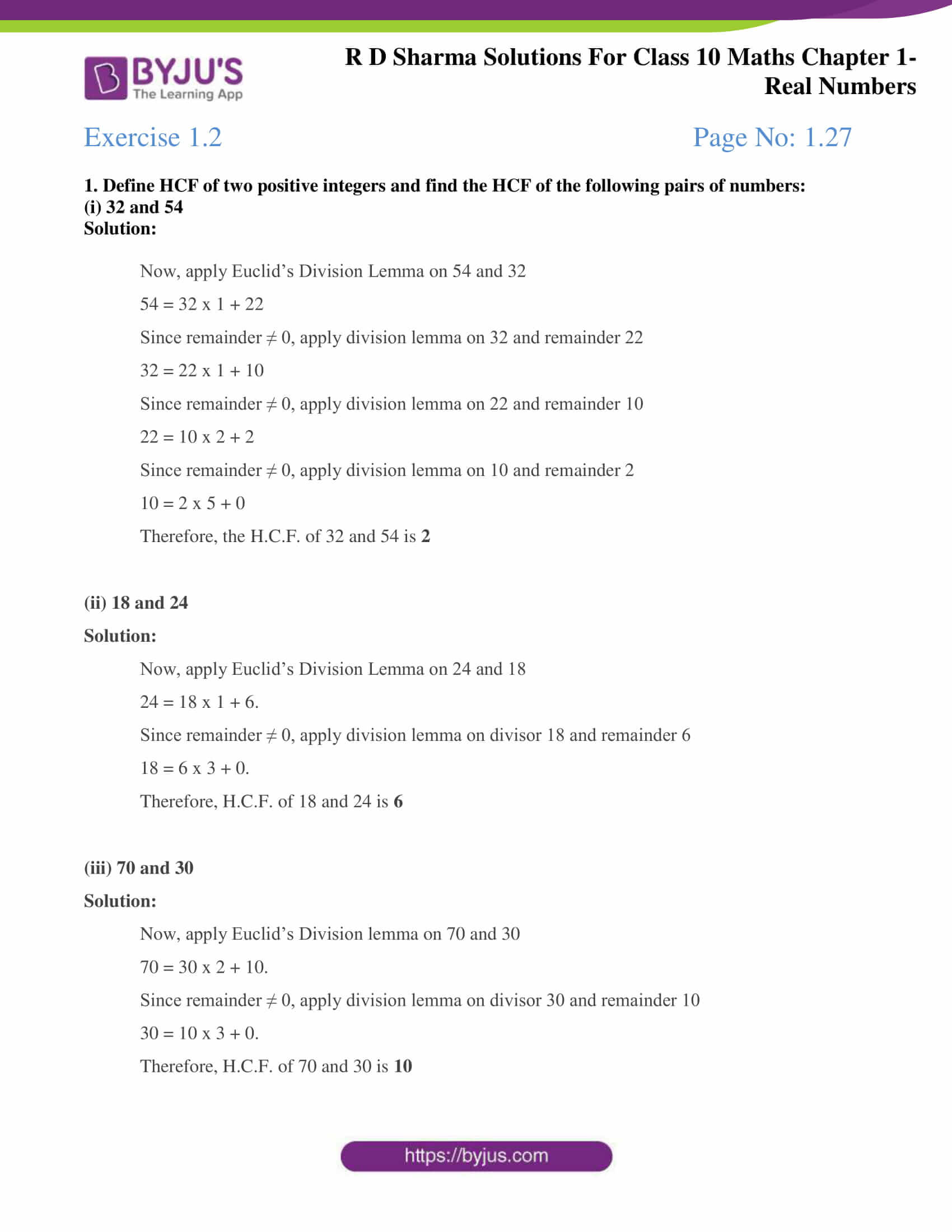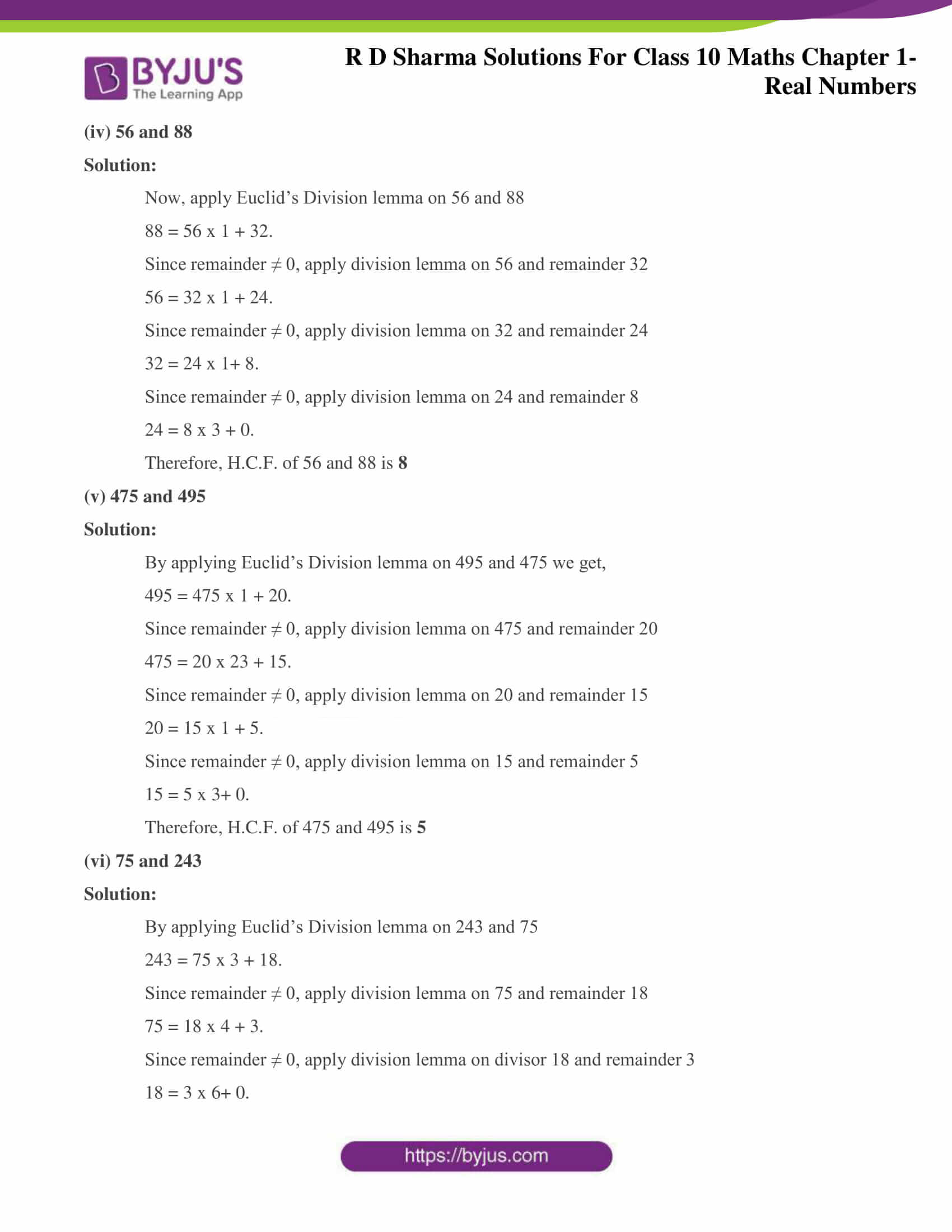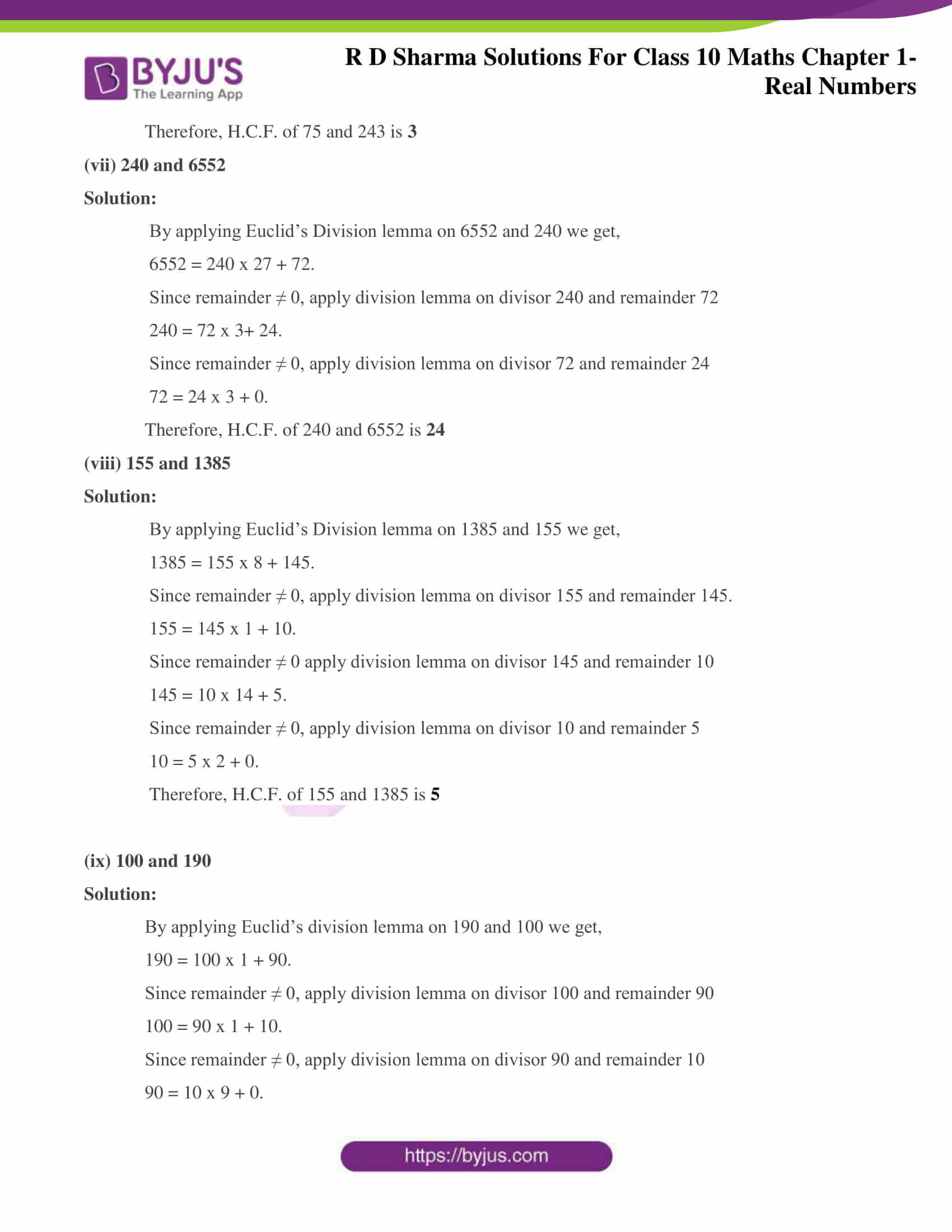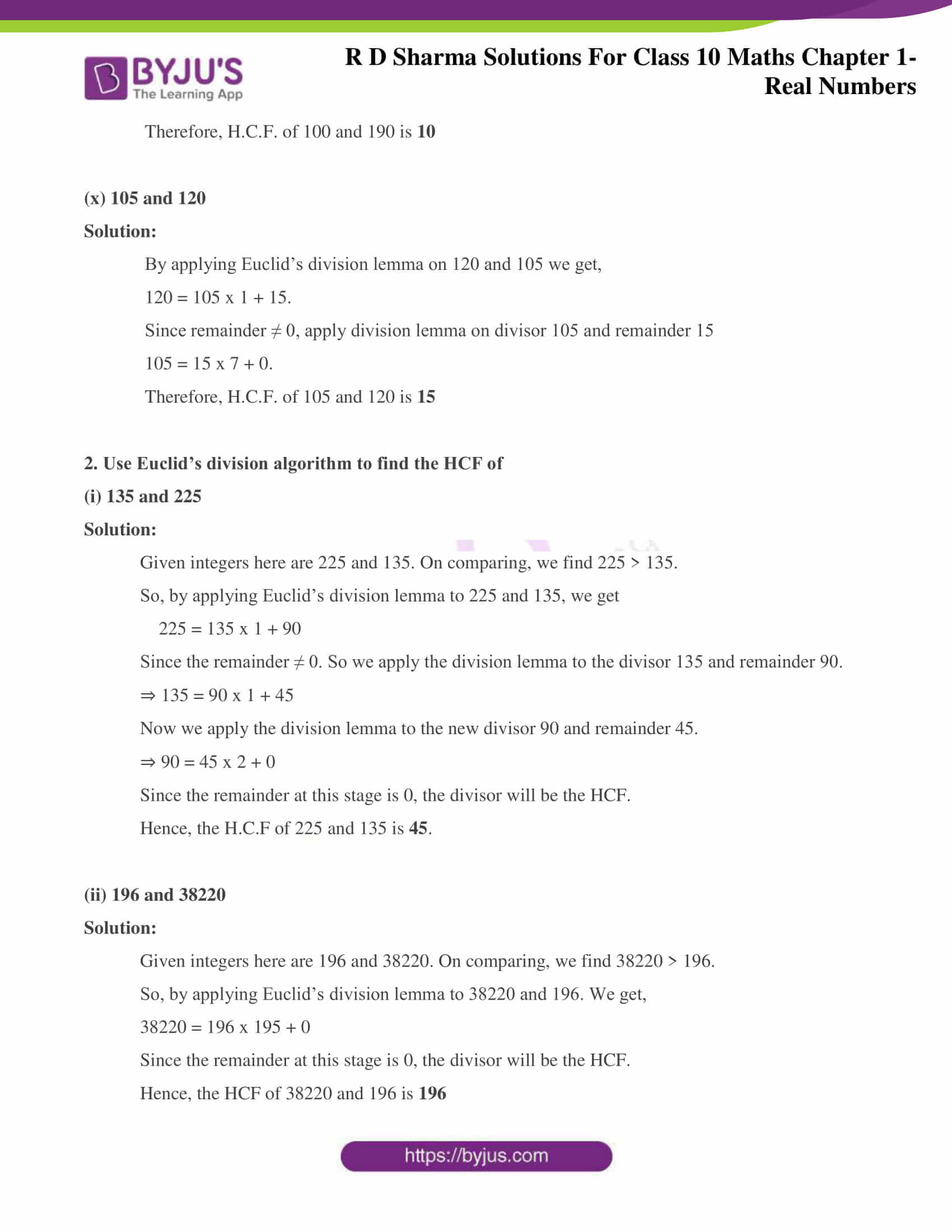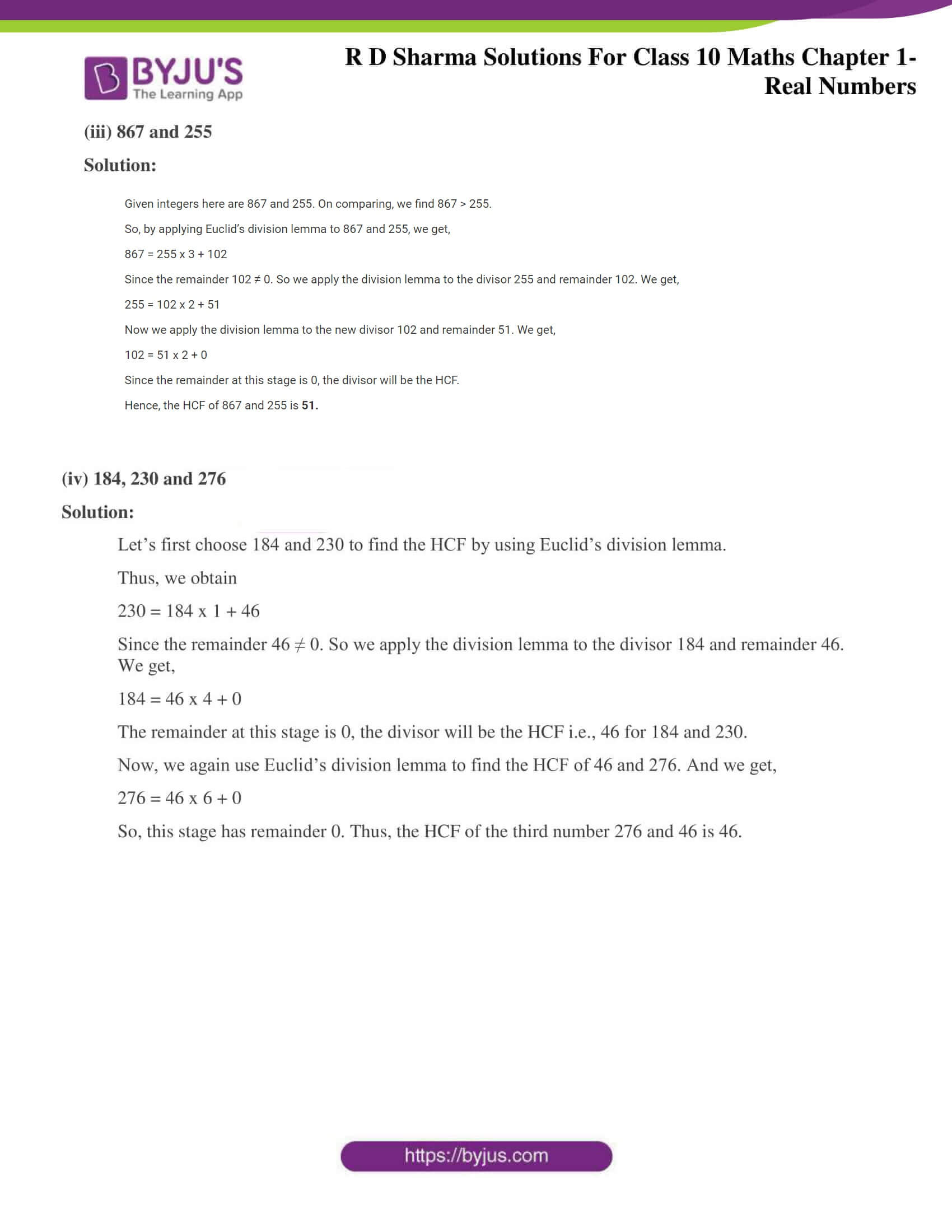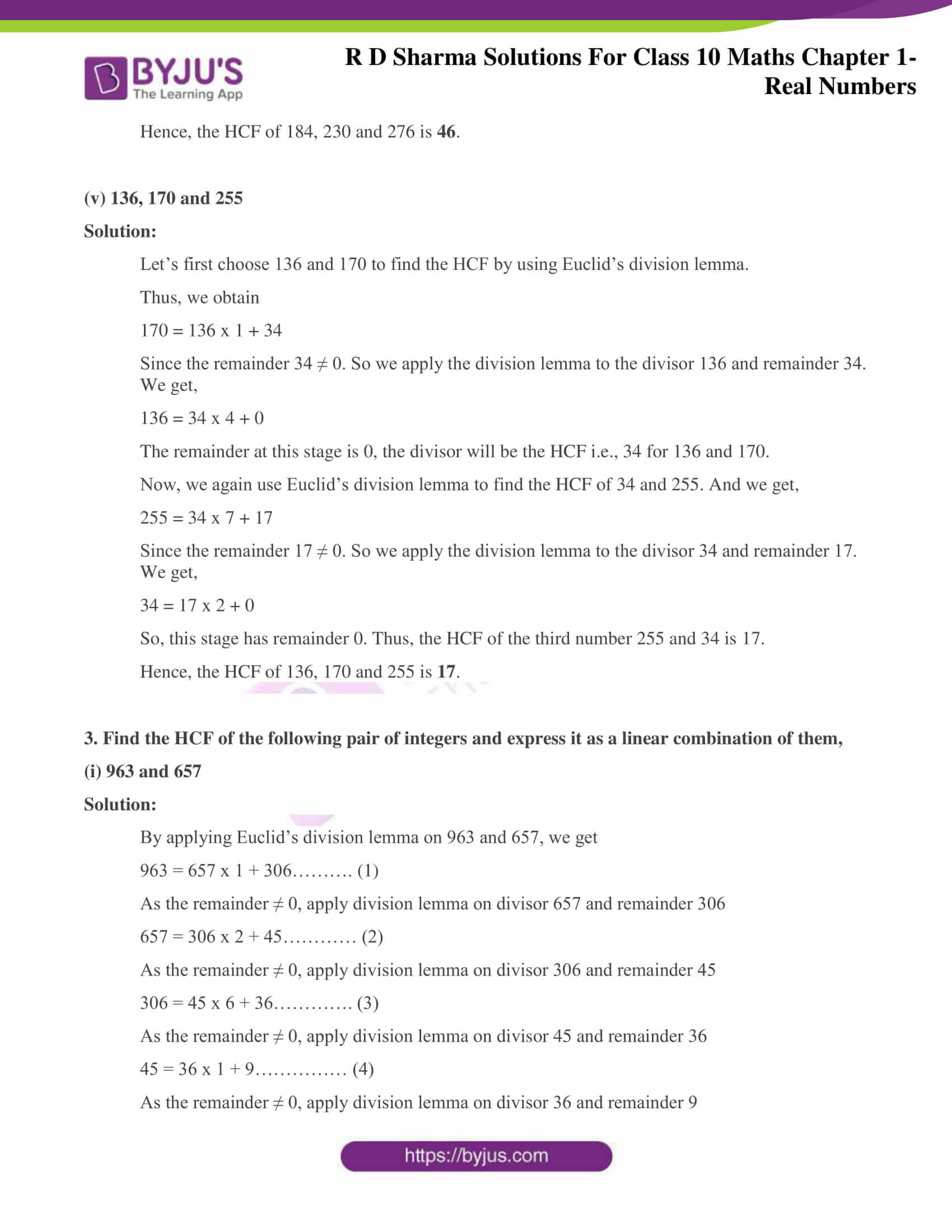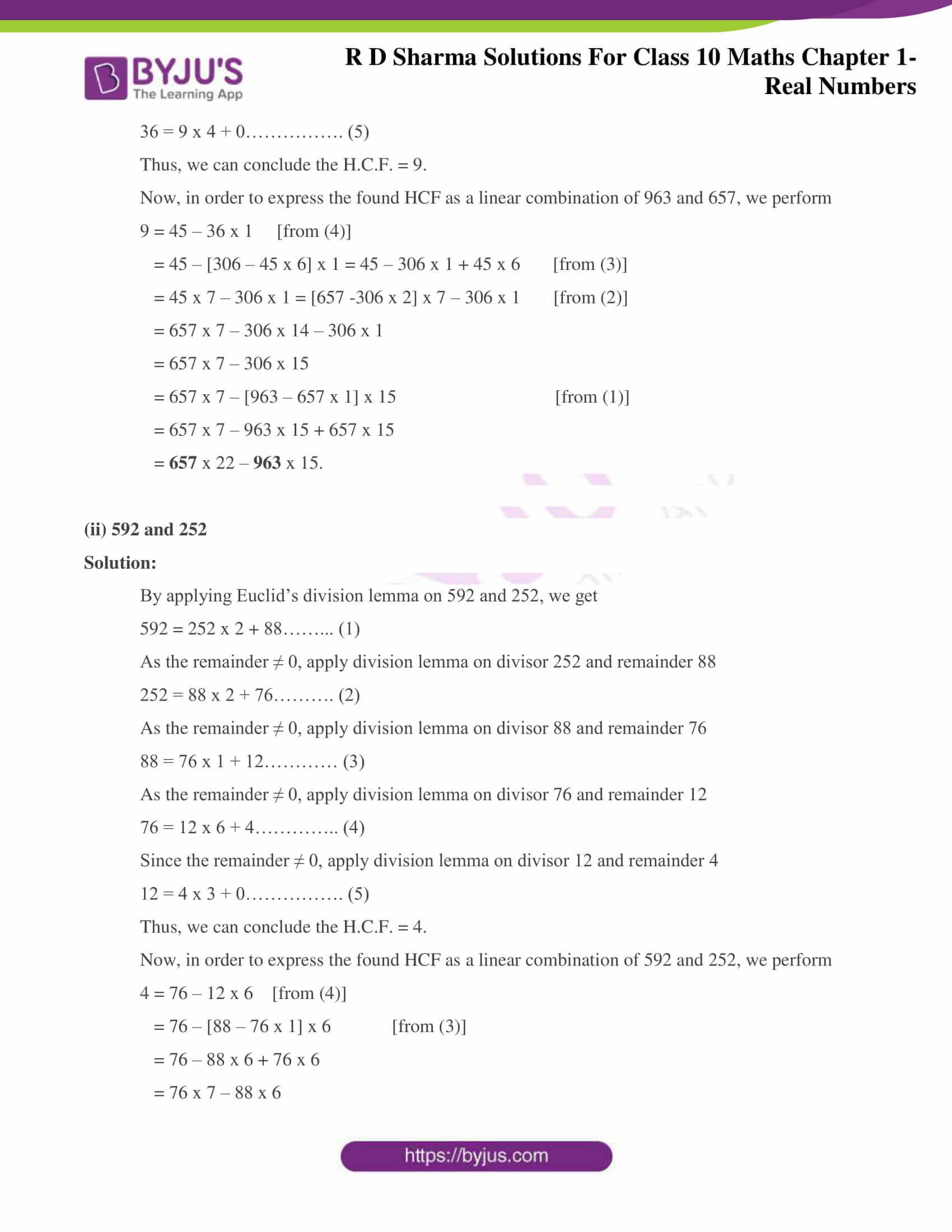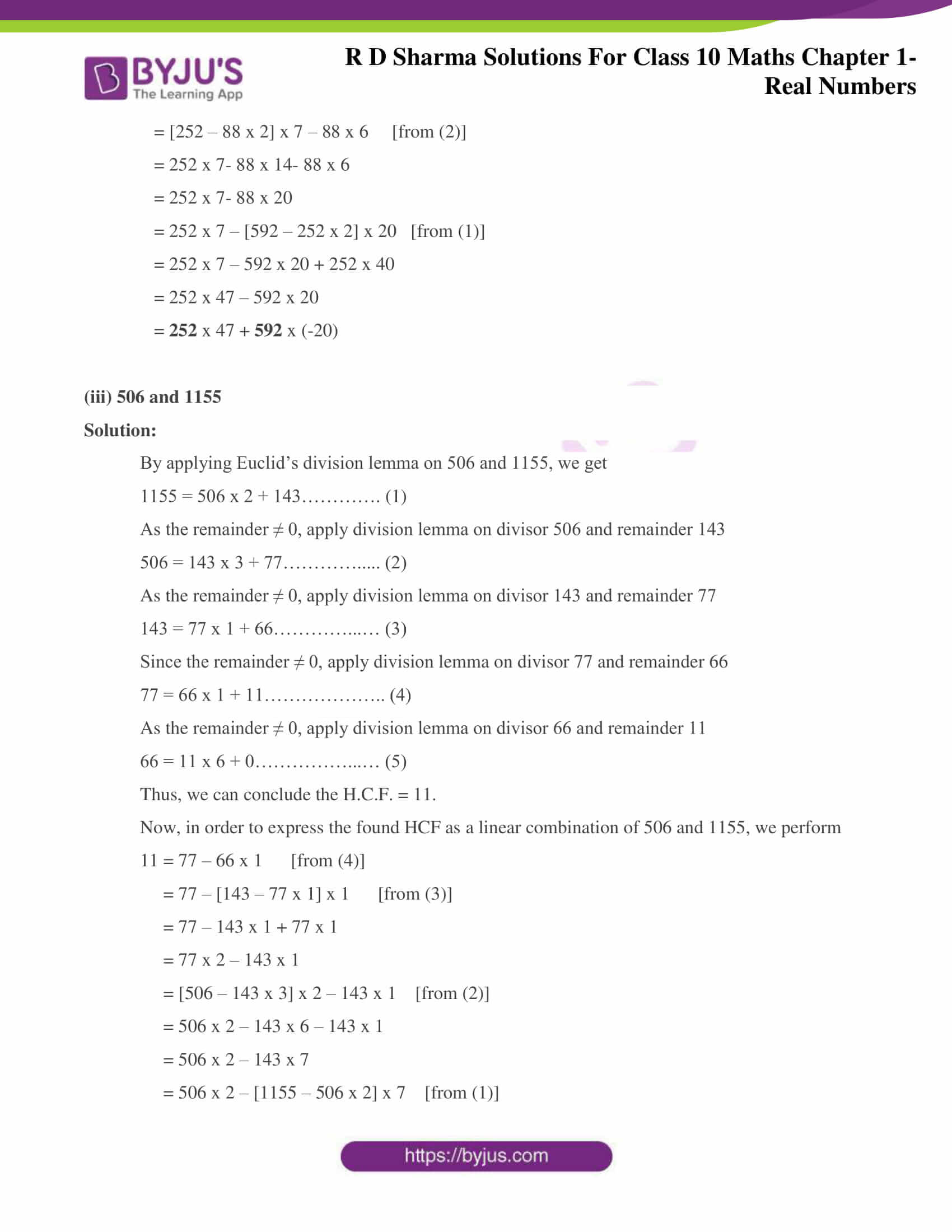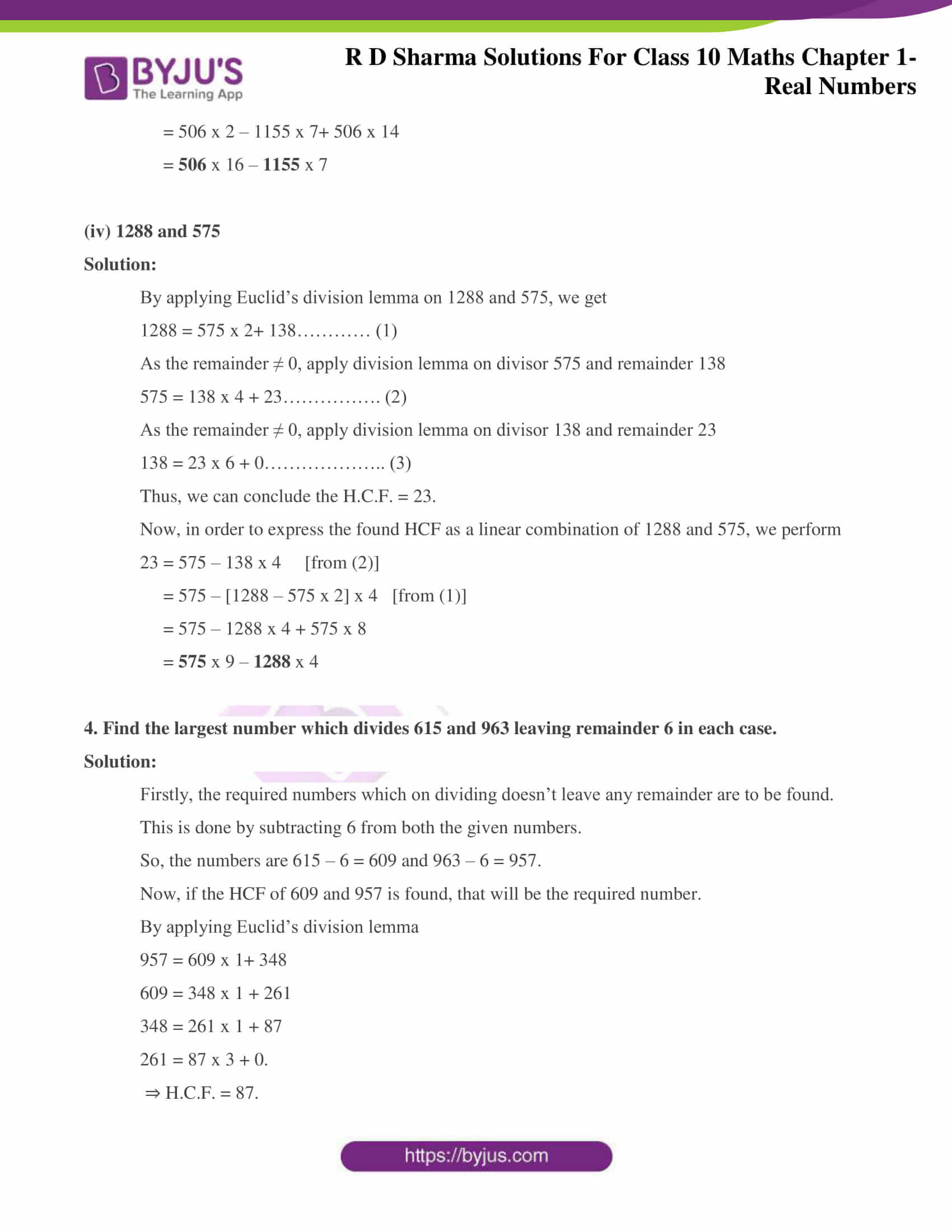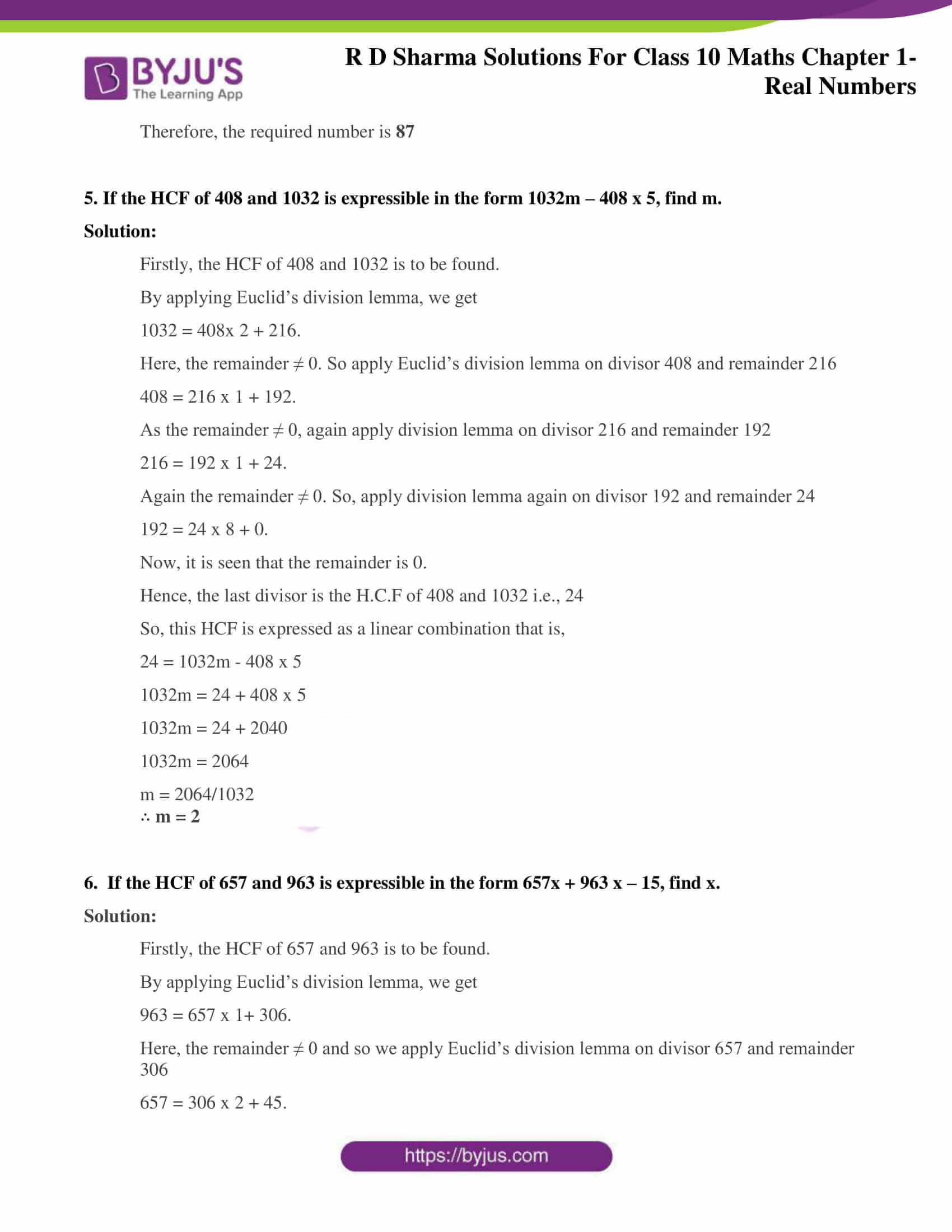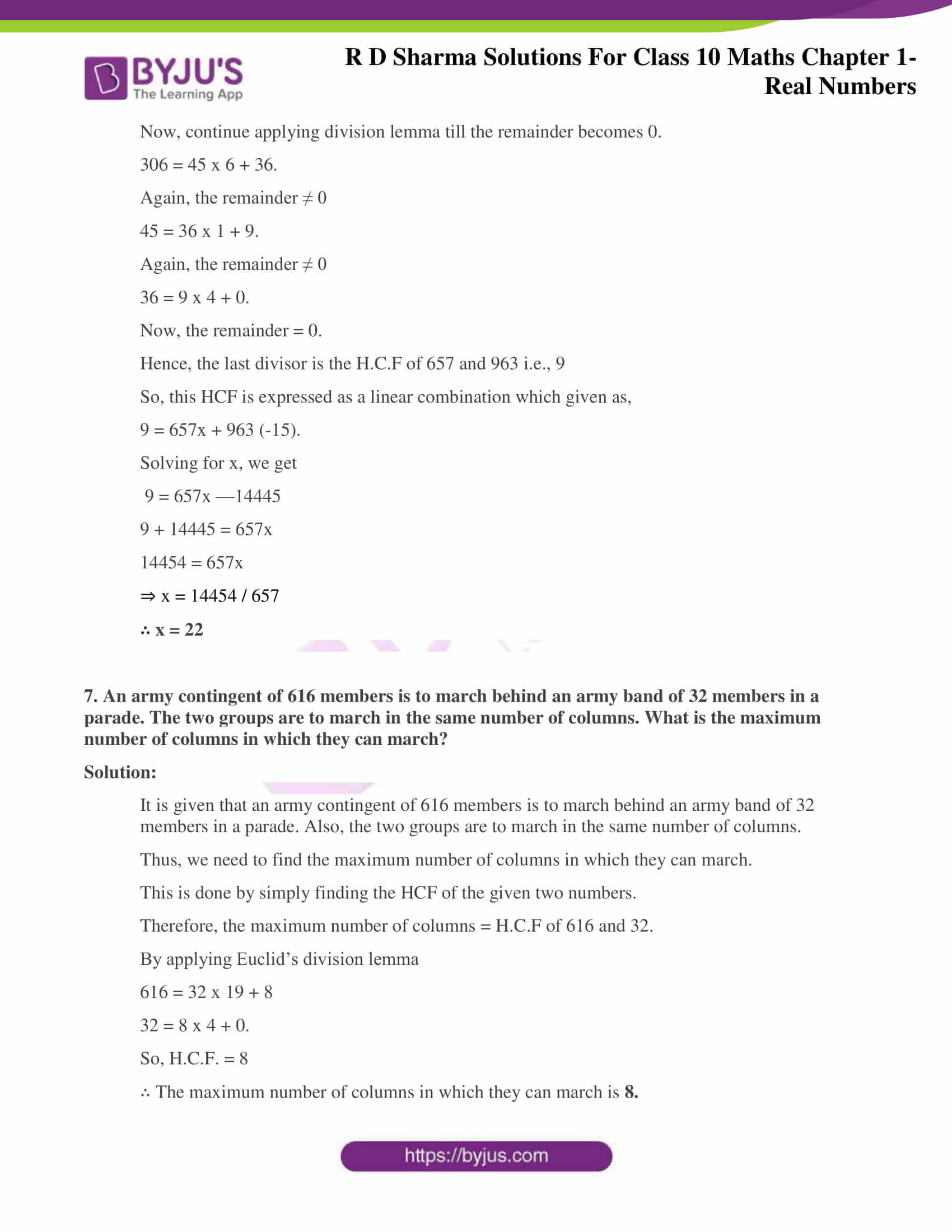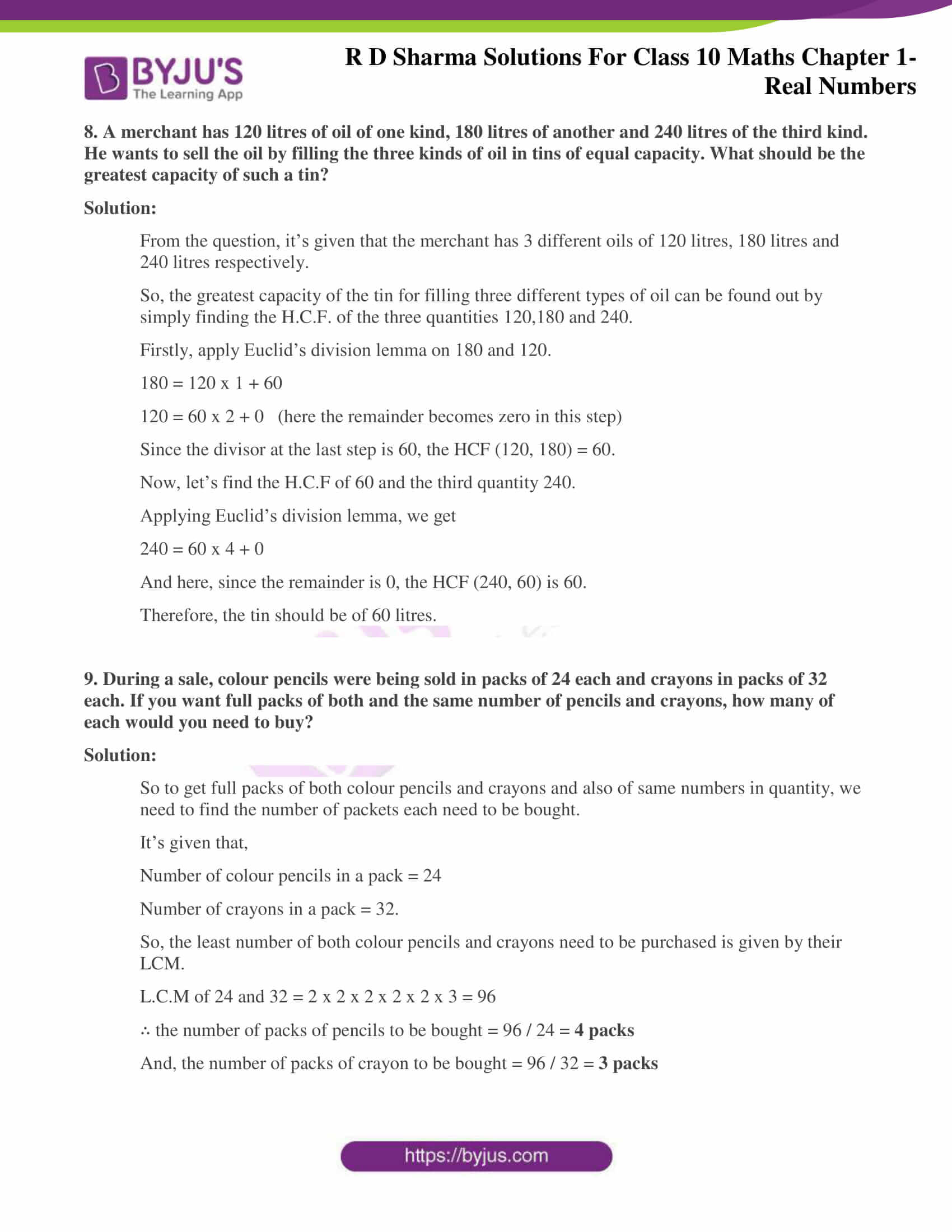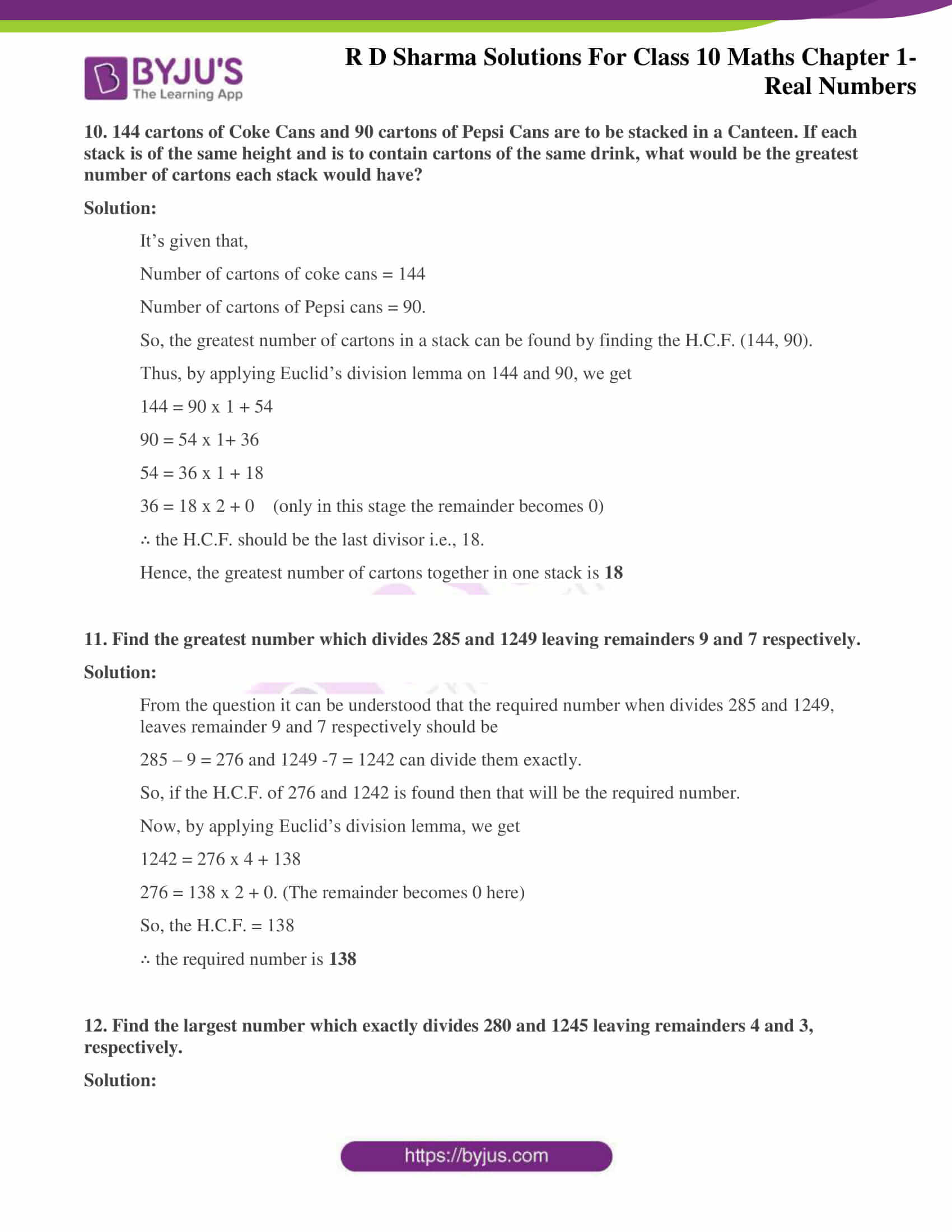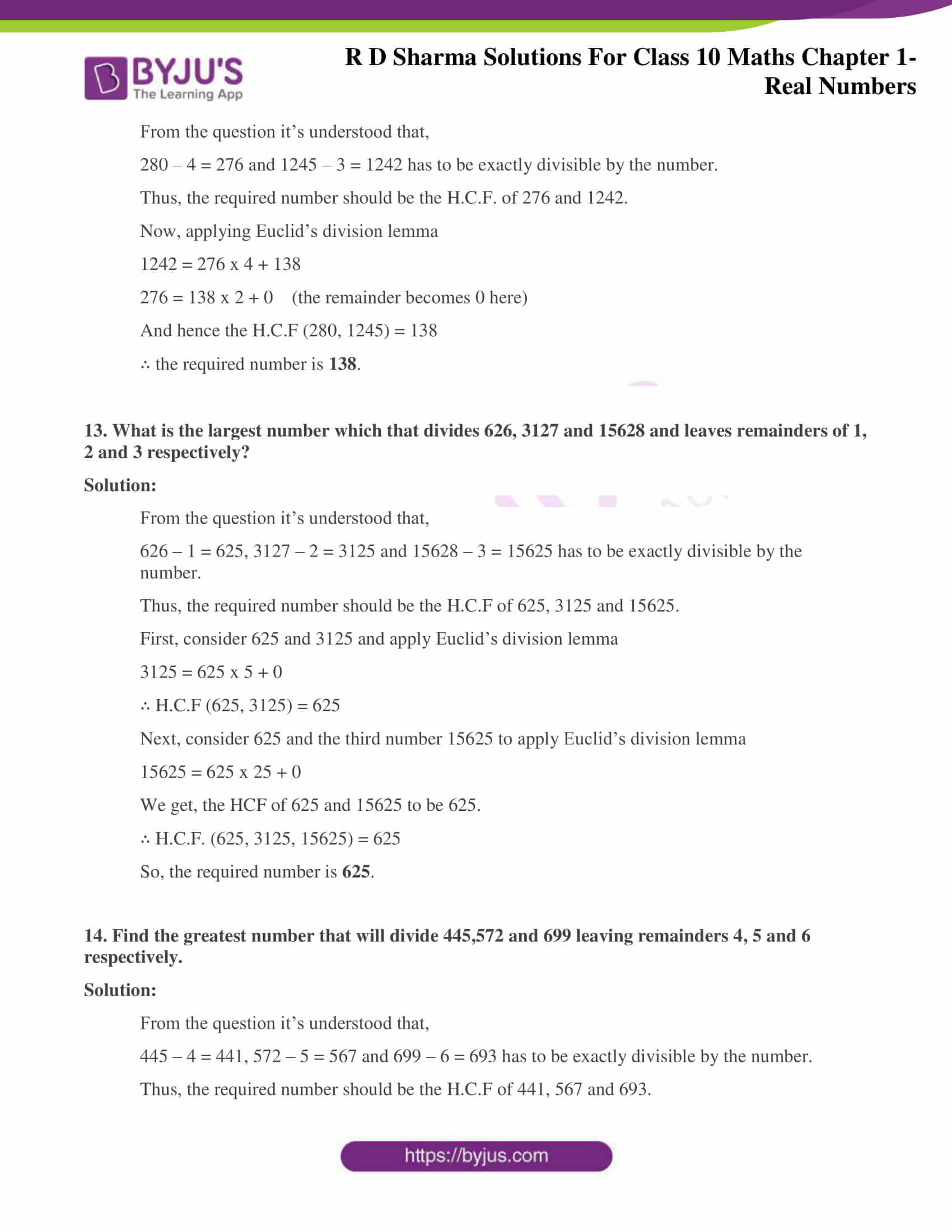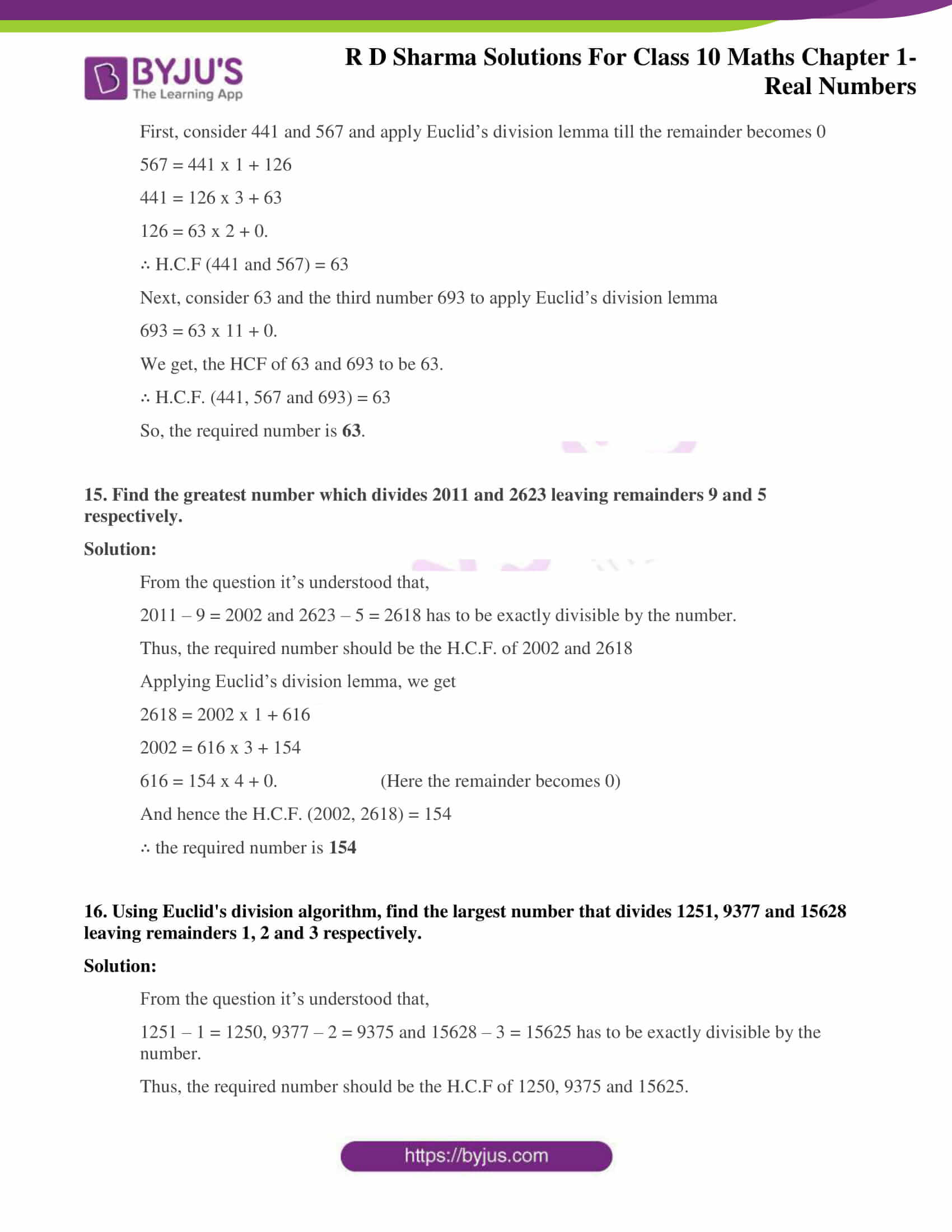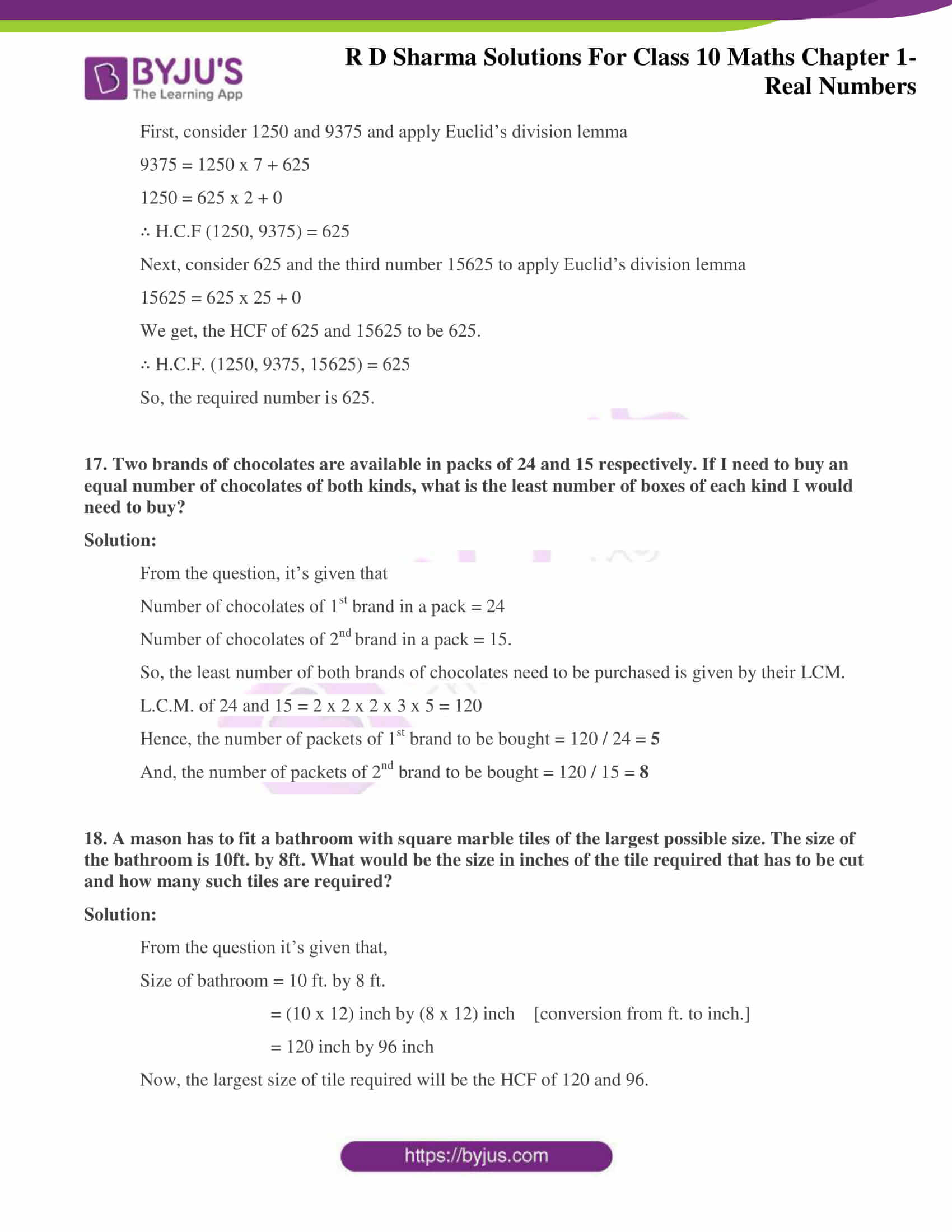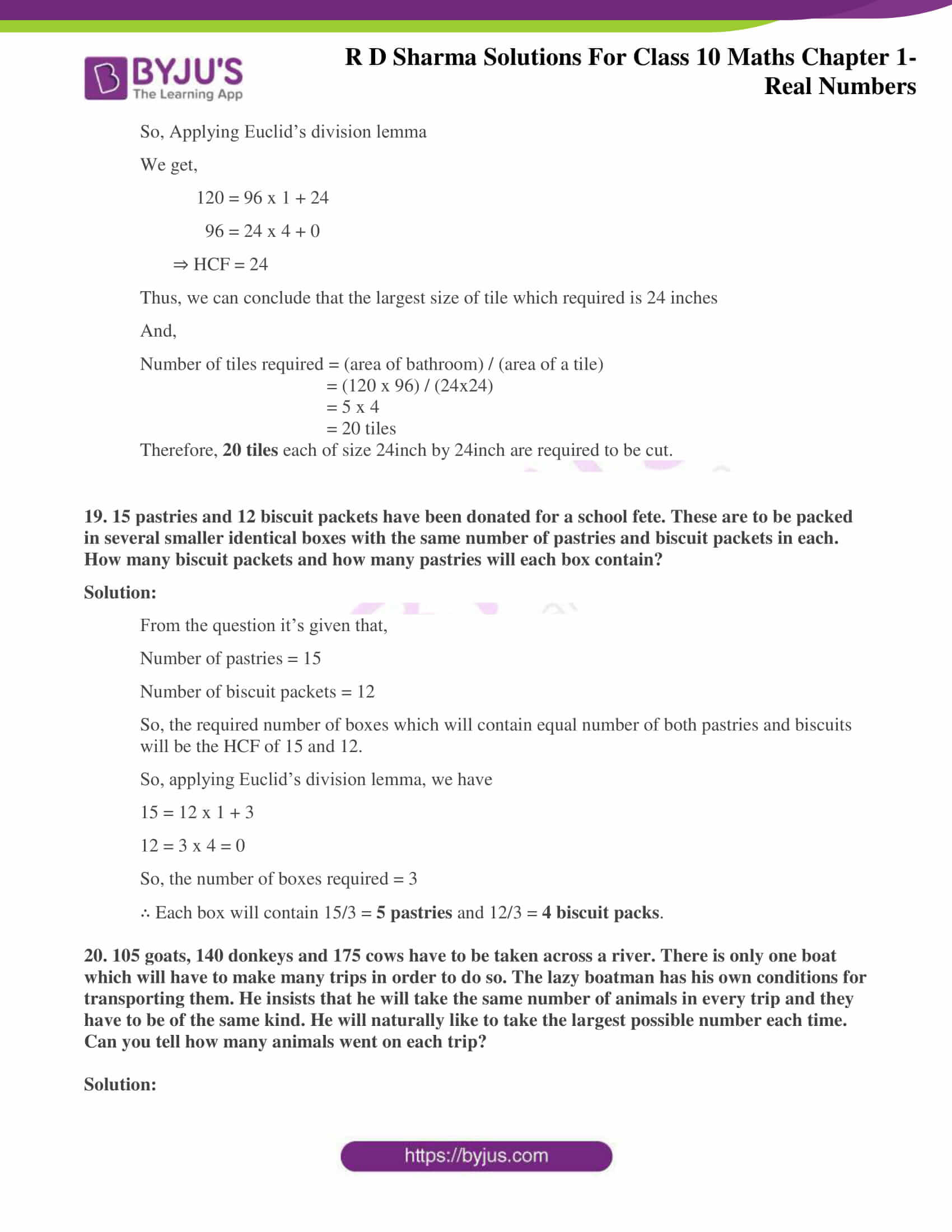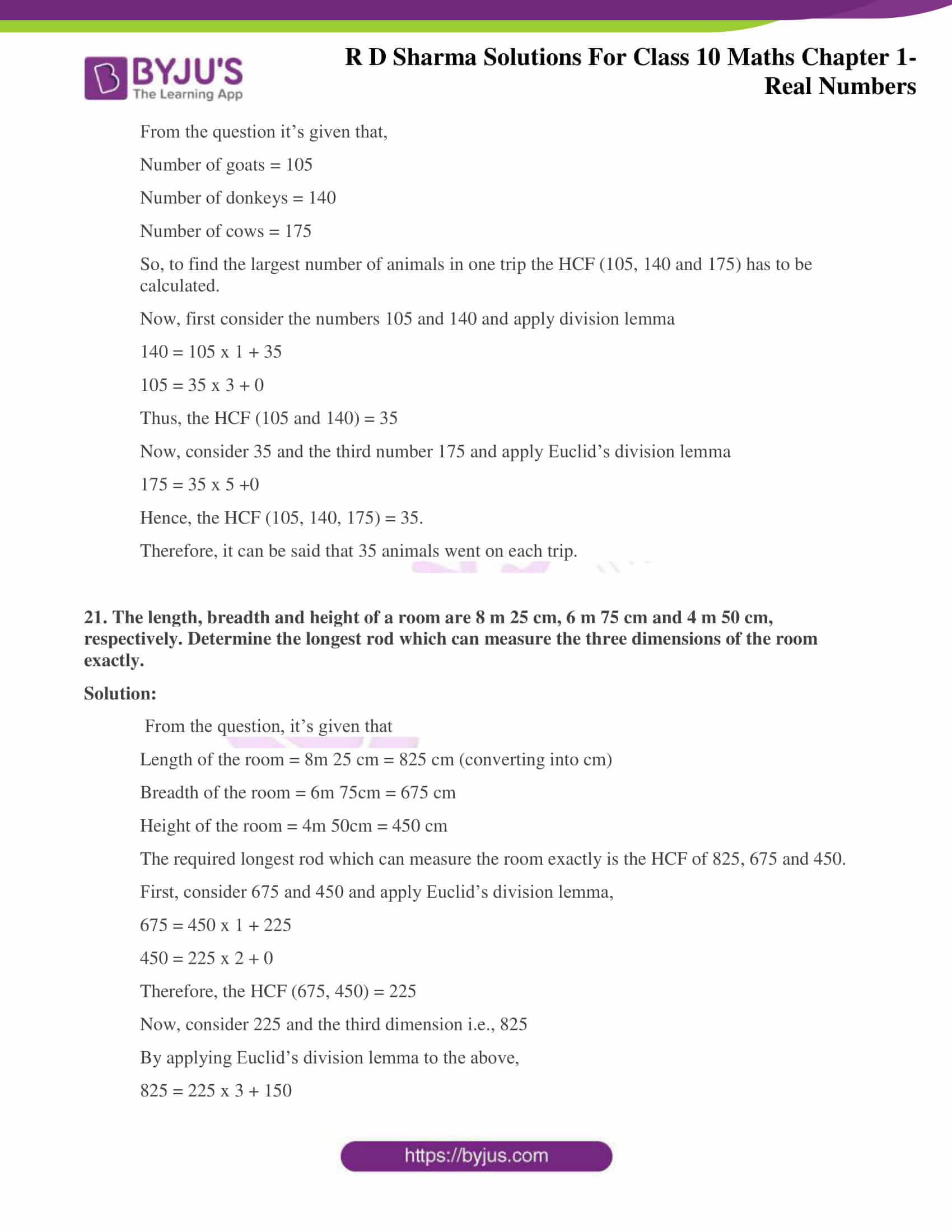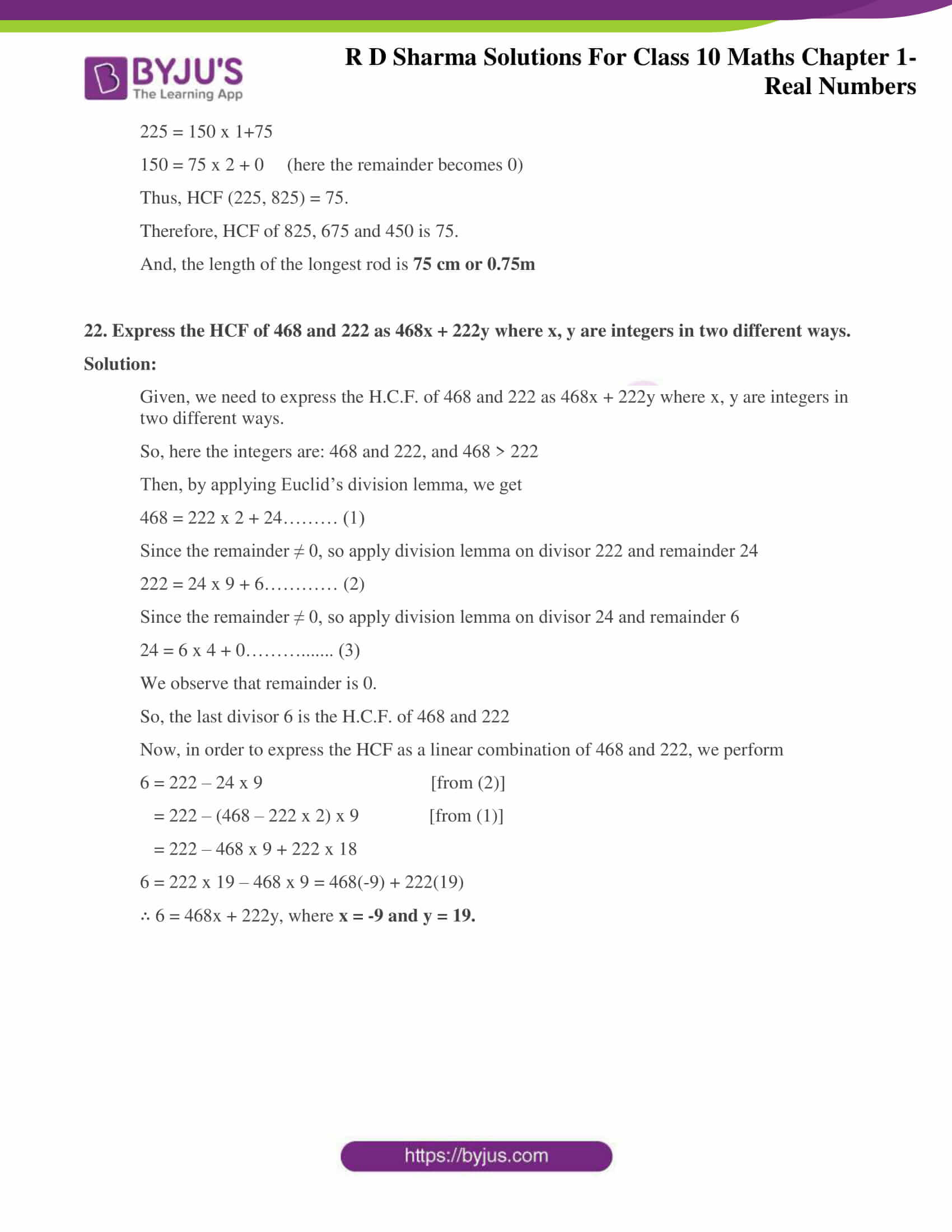### Access answers to Maths RD Sharma Solutions for Class 10 Chapter 1 Real Numbers Exercise 1.2

1. Define HCF of two positive integers and find the HCF of the following pairs of numbers:

(i) 32 and 54

Solution:

Now, apply Euclid’s Division Lemma on 54 and 32

54 = 32 x 1 + 22

Since remainder ≠ 0, apply division lemma on 32 and remainder 22

32 = 22 x 1 + 10

Since remainder ≠ 0, apply division lemma on 22 and remainder 10

22 = 10 x 2 + 2

Since remainder ≠ 0, apply division lemma on 10 and remainder 2

10 = 2 x 5 + 0

Therefore, the H.C.F. of 32 and 54 is 2

(ii) 18 and 24

Solution:

Now, apply Euclid’s Division Lemma on 24 and 18

24 = 18 x 1 + 6.

Since remainder ≠ 0, apply division lemma on divisor 18 and remainder 6

18 = 6 x 3 + 0.

Therefore, H.C.F. of 18 and 24 is 6

(iii) 70 and 30

Solution:

Now, apply Euclid’s Division lemma on 70 and 30

70 = 30 x 2 + 10.

Since remainder ≠ 0, apply division lemma on divisor 30 and remainder 10

30 = 10 x 3 + 0.

Therefore, H.C.F. of 70 and 30 is 10

(iv) 56 and 88

Solution:

Now, apply Euclid’s Division lemma on 56 and 88

88 = 56 x 1 + 32.

Since remainder ≠ 0, apply division lemma on 56 and remainder 32

56 = 32 x 1 + 24.

Since remainder ≠ 0, apply division lemma on 32 and remainder 24

32 = 24 x 1+ 8.

Since remainder ≠ 0, apply division lemma on 24 and remainder 8

24 = 8 x 3 + 0.

Therefore, H.C.F. of 56 and 88 is 8

(v) 475 and 495

Solution:

By applying Euclid’s Division lemma on 495 and 475 we get,

495 = 475 x 1 + 20.

Since remainder ≠ 0, apply division lemma on 475 and remainder 20

475 = 20 x 23 + 15.

Since remainder ≠ 0, apply division lemma on 20 and remainder 15

20 = 15 x 1 + 5.

Since remainder ≠ 0, apply division lemma on 15 and remainder 5

15 = 5 x 3+ 0.

Therefore, H.C.F. of 475 and 495 is 5

(vi) 75 and 243

Solution:

By applying Euclid’s Division lemma on 243 and 75

243 = 75 x 3 + 18.

Since remainder ≠ 0, apply division lemma on 75 and remainder 18

75 = 18 x 4 + 3.

Since remainder ≠ 0, apply division lemma on divisor 18 and remainder 3

18 = 3 x 6+ 0.

Therefore, H.C.F. of 75 and 243 is 3

(vii) 240 and 6552

Solution:

By applying Euclid’s Division lemma on 6552 and 240 we get,

6552 = 240 x 27 + 72.

Since remainder ≠ 0, apply division lemma on divisor 240 and remainder 72

240 = 72 x 3+ 24.

Since remainder ≠ 0, apply division lemma on divisor 72 and remainder 24

72 = 24 x 3 + 0.

Therefore, H.C.F. of 240 and 6552 is 24

(viii) 155 and 1385

Solution:

By applying Euclid’s Division lemma on 1385 and 155 we get,

1385 = 155 x 8 + 145.

Since remainder ≠ 0, apply division lemma on divisor 155 and remainder 145.

155 = 145 x 1 + 10.

Since remainder ≠ 0 apply division lemma on divisor 145 and remainder 10

145 = 10 x 14 + 5.

Since remainder ≠ 0, apply division lemma on divisor 10 and remainder 5

10 = 5 x 2 + 0.

Therefore, H.C.F. of 155 and 1385 is 5

(ix) 100 and 190

Solution:

By applying Euclid’s division lemma on 190 and 100 we get,

190 = 100 x 1 + 90.

Since remainder ≠ 0, apply division lemma on divisor 100 and remainder 90

100 = 90 x 1 + 10.

Since remainder ≠ 0, apply division lemma on divisor 90 and remainder 10

90 = 10 x 9 + 0.

Therefore, H.C.F. of 100 and 190 is 10

(x) 105 and 120

Solution:

By applying Euclid’s division lemma on 120 and 105 we get,

120 = 105 x 1 + 15.

Since remainder ≠ 0, apply division lemma on divisor 105 and remainder 15

105 = 15 x 7 + 0.

Therefore, H.C.F. of 105 and 120 is 15

2. Use Euclid’s division algorithm to find the HCF of

(i) 135 and 225

Solution:

Given integers here are 225 and 135. On comparing, we find 225 > 135.

So, by applying Euclid’s division lemma to 225 and 135, we get

225 = 135 x 1 + 90

Since the remainder ≠ 0. So we apply the division lemma to the divisor 135 and remainder 90.

⇒ 135 = 90 x 1 + 45

Now we apply the division lemma to the new divisor 90 and remainder 45.

⇒ 90 = 45 x 2 + 0

Since the remainder at this stage is 0, the divisor will be the HCF.

Hence, the H.C.F of 225 and 135 is 45.

(ii) 196 and 38220

Solution:

Given integers here are 196 and 38220. On comparing, we find 38220 > 196.

So, by applying Euclid’s division lemma to 38220 and 196. We get,

38220 = 196 x 195 + 0

Since the remainder at this stage is 0, the divisor will be the HCF.

Hence, the HCF of 38220 and 196 is 196

(iii) 867 and 255

Solution:

Given integers here are 867 and 255. On comparing, we find 867 > 255.

So, by applying Euclid’s division lemma to 867 and 255, we get,

867 = 255 x 3 + 102

Since the remainder 102 ≠ 0. So we apply the division lemma to the divisor 255 and remainder 102. We get,

255 = 102 x 2 + 51

Now we apply the division lemma to the new divisor 102 and remainder 51. We get,

102 = 51 x 2 + 0

Since the remainder at this stage is 0, the divisor will be the HCF.

Hence, the HCF of 867 and 255 is 51.

(iv) 184, 230 and 276

Solution:

Let’s first choose 184 and 230 to find the HCF by using Euclid’s division lemma.

Thus, we obtain

230 = 184 x 1 + 46

Since the remainder 46 ≠ 0. So we apply the division lemma to the divisor 184 and remainder 46. We get,

184 = 46 x 4 + 0

The remainder at this stage is 0, the divisor will be the HCF i.e., 46 for 184 and 230.

Now, we again use Euclid’s division lemma to find the HCF of 46 and 276. And we get,

276 = 46 x 6 + 0

So, this stage has remainder 0. Thus, the HCF of the third number 276 and 46 is 46.

Hence, the HCF of 184, 230 and 276 is 46.

(v) 136, 170 and 255

Solution:

Let’s first choose 136 and 170 to find the HCF by using Euclid’s division lemma.

Thus, we obtain

170 = 136 x 1 + 34

Since the remainder 34 ≠ 0. So we apply the division lemma to the divisor 136 and remainder 34. We get,

136 = 34 x 4 + 0

The remainder at this stage is 0, the divisor will be the HCF i.e., 34 for 136 and 170.

Now, we again use Euclid’s division lemma to find the HCF of 34 and 255. And we get,

255 = 34 x 7 + 17

Since the remainder 17 ≠ 0. So we apply the division lemma to the divisor 34 and remainder 17. We get,

34 = 17 x 2 + 0

So, this stage has remainder 0. Thus, the HCF of the third number 255 and 34 is 17.

Hence, the HCF of 136, 170 and 255 is 17.

3. Find the HCF of the following pair of integers and express it as a linear combination of them,

(i) 963 and 657

Solution:

By applying Euclid’s division lemma on 963 and 657, we get

963 = 657 x 1 + 306………. (1)

As the remainder ≠ 0, apply division lemma on divisor 657 and remainder 306

657 = 306 x 2 + 45………… (2)

As the remainder ≠ 0, apply division lemma on divisor 306 and remainder 45

306 = 45 x 6 + 36…………. (3)

As the remainder ≠ 0, apply division lemma on divisor 45 and remainder 36

45 = 36 x 1 + 9…………… (4)

As the remainder ≠ 0, apply division lemma on divisor 36 and remainder 9

36 = 9 x 4 + 0……………. (5)

Thus, we can conclude the H.C.F. = 9.

Now, in order to express the found HCF as a linear combination of 963 and 657, we perform

9 = 45 – 36 x 1 [from (4)]

= 45 – [306 – 45 x 6] x 1 = 45 – 306 x 1 + 45 x 6 [from (3)]

= 45 x 7 – 306 x 1 = [657 -306 x 2] x 7 – 306 x 1 [from (2)]

= 657 x 7 – 306 x 14 – 306 x 1

= 657 x 7 – 306 x 15

= 657 x 7 – [963 – 657 x 1] x 15 [from (1)]

= 657 x 7 – 963 x 15 + 657 x 15

657 x 22 – 963 x 15.

(ii) 592 and 252

Solution:

By applying Euclid’s division lemma on 592 and 252, we get

592 = 252 x 2 + 88……… (1)

As the remainder ≠ 0, apply division lemma on divisor 252 and remainder 88

252 = 88 x 2 + 76………. (2)

As the remainder ≠ 0, apply division lemma on divisor 88 and remainder 76

88 = 76 x 1 + 12………… (3)

As the remainder ≠ 0, apply division lemma on divisor 76 and remainder 12

76 = 12 x 6 + 4………….. (4)

Since the remainder ≠ 0, apply division lemma on divisor 12 and remainder 4

12 = 4 x 3 + 0……………. (5)

Thus, we can conclude the H.C.F. = 4.

Now, in order to express the found HCF as a linear combination of 592 and 252, we perform

4 = 76 – 12 x 6 [from (4)]

= 76 – [88 – 76 x 1] x 6 [from (3)]

= 76 – 88 x 6 + 76 x 6

= 76 x 7 – 88 x 6

= [252 – 88 x 2] x 7 – 88 x 6 [from (2)]

= 252 x 7- 88 x 14- 88 x 6

= 252 x 7- 88 x 20

= 252 x 7 – [592 – 252 x 2] x 20 [from (1)]

= 252 x 7 – 592 x 20 + 252 x 40

= 252 x 47 – 592 x 20

252 x 47 + 592 x (-20)

(iii) 506 and 1155

Solution:

By applying Euclid’s division lemma on 506 and 1155, we get

1155 = 506 x 2 + 143…………. (1)

As the remainder ≠ 0, apply division lemma on divisor 506 and remainder 143

506 = 143 x 3 + 77…………….. (2)

As the remainder ≠ 0, apply division lemma on divisor 143 and remainder 77

143 = 77 x 1 + 66……………… (3)

Since the remainder ≠ 0, apply division lemma on divisor 77 and remainder 66

77 = 66 x 1 + 11……………….. (4)

As the remainder ≠ 0, apply division lemma on divisor 66 and remainder 11

66 = 11 x 6 + 0………………… (5)

Thus, we can conclude the H.C.F. = 11.

Now, in order to express the found HCF as a linear combination of 506 and 1155, we perform

11 = 77 – 66 x 1 [from (4)]

= 77 – [143 – 77 x 1] x 1 [from (3)]

= 77 – 143 x 1 + 77 x 1

= 77 x 2 – 143 x 1

= [506 – 143 x 3] x 2 – 143 x 1 [from (2)]

= 506 x 2 – 143 x 6 – 143 x 1

= 506 x 2 – 143 x 7

= 506 x 2 – [1155 – 506 x 2] x 7 [from (1)]

= 506 x 2 – 1155 x 7+ 506 x 14

506 x 16 – 1155 x 7

(iv) 1288 and 575

Solution:

By applying Euclid’s division lemma on 1288 and 575, we get

1288 = 575 x 2+ 138………… (1)

As the remainder ≠ 0, apply division lemma on divisor 575 and remainder 138

575 = 138 x 4 + 23……………. (2)

As the remainder ≠ 0, apply division lemma on divisor 138 and remainder 23

138 = 23 x 6 + 0……………….. (3)

Thus, we can conclude the H.C.F. = 23.

Now, in order to express the found HCF as a linear combination of 1288 and 575, we perform

23 = 575 – 138 x 4 [from (2)]

= 575 – [1288 – 575 x 2] x 4 [from (1)]

= 575 – 1288 x 4 + 575 x 8

= 575 x 9 – 1288 x 4

4. Find the largest number which divides 615 and 963 leaving remainder 6 in each case.

Solution:

Firstly, the required numbers which on dividing doesn’t leave any remainder are to be found.

This is done by subtracting 6 from both the given numbers.

So, the numbers are 615 – 6 = 609 and 963 – 6 = 957.

Now, if the HCF of 609 and 957 is found, that will be the required number.

By applying Euclid’s division lemma

957 = 609 x 1+ 348

609 = 348 x 1 + 261

348 = 261 x 1 + 87

261 = 87 x 3 + 0.

⇒ H.C.F. = 87.

Therefore, the required number is 87

5. If the HCF of 408 and 1032 is expressible in the form 1032m – 408 x 5, find m.

Solution:

Firstly, the HCF of 408 and 1032 is to be found.

By applying Euclid’s division lemma, we get

1032 = 408x 2 + 216.

Here, the remainder ≠ 0. So apply Euclid’s division lemma on divisor 408 and remainder 216

408 = 216 x 1 + 192.

As the remainder ≠ 0, again apply division lemma on divisor 216 and remainder 192

216 = 192 x 1 + 24.

Again the remainder ≠ 0. So, apply division lemma again on divisor 192 and remainder 24

192 = 24 x 8 + 0.

Now, it is seen that the remainder is 0.

Hence, the last divisor is the H.C.F of 408 and 1032 i.e., 24

So, this HCF is expressed as a linear combination that is,

24 = 1032m – 408 x 5

1032m = 24 + 408 x 5

1032m = 24 + 2040

1032m = 2064

m = 2064/1032

m = 2

6.  If the HCF of 657 and 963 is expressible in the form 657x + 963 x – 15, find x.

Solution:

Firstly, the HCF of 657 and 963 is to be found.

By applying Euclid’s division lemma, we get

963 = 657 x 1+ 306.

Here, the remainder ≠ 0 and so we apply Euclid’s division lemma on divisor 657 and remainder 306

657 = 306 x 2 + 45.

Now, continue applying division lemma till the remainder becomes 0.

306 = 45 x 6 + 36.

Again, the remainder ≠ 0

45 = 36 x 1 + 9.

Again, the remainder ≠ 0

36 = 9 x 4 + 0.

Now, the remainder = 0.

Hence, the last divisor is the H.C.F of 657 and 963 i.e., 9

So, this HCF is expressed as a linear combination which given as,

9 = 657x + 963 (-15).

Solving for x, we get

9 = 657x —14445

9 + 14445 = 657x

14454 = 657x

⇒ x = 14454 / 657

∴ x = 22

7. An army contingent of 616 members is to march behind an army band of 32 members in a parade. The two groups are to march in the same number of columns. What is the maximum number of columns in which they can march?

Solution:

It is given that an army contingent of 616 members is to march behind an army band of 32 members in a parade. Also, the two groups are to march in the same number of columns.

Thus, we need to find the maximum number of columns in which they can march.

This is done by simply finding the HCF of the given two numbers.

Therefore, the maximum number of columns = H.C.F of 616 and 32.

By applying Euclid’s division lemma

616 = 32 x 19 + 8

32 = 8 x 4 + 0.

So, H.C.F. = 8

∴ The maximum number of columns in which they can march is 8.

8. A merchant has 120 litres of oil of one kind, 180 litres of another and 240 litres of the third kind. He wants to sell the oil by filling the three kinds of oil in tins of equal capacity. What should be the greatest capacity of such a tin?

Solution:

From the question, it’s given that the merchant has 3 different oils of 120 litres, 180 litres and 240 litres respectively.

So, the greatest capacity of the tin for filling three different types of oil can be found out by simply finding the H.C.F. of the three quantities 120,180 and 240.

Firstly, apply Euclid’s division lemma on 180 and 120.

180 = 120 x 1 + 60

120 = 60 x 2 + 0 (here the remainder becomes zero in this step)

Since the divisor at the last step is 60, the HCF (120, 180) = 60.

Now, let’s find the H.C.F of 60 and the third quantity 240.

Applying Euclid’s division lemma, we get

240 = 60 x 4 + 0

And here, since the remainder is 0, the HCF (240, 60) is 60.

Therefore, the tin should be of 60 litres.

9. During a sale, colour pencils were being sold in packs of 24 each and crayons in packs of 32 each. If you want full packs of both and the same number of pencils and crayons, how many of each would you need to buy?

Solution:

So to get full packs of both colour pencils and crayons and also of same numbers in quantity, we need to find the number of packets each need to be bought.

It’s given that,

Number of colour pencils in a pack = 24

Number of crayons in a pack = 32.

So, the least number of both colour pencils and crayons need to be purchased is given by their LCM.

L.C.M of 24 and 32 = 2 x 2 x 2 x 2 x 2 x 3 = 96

∴ the number of packs of pencils to be bought = 96 / 24 = 4 packs

And, the number of packs of crayon to be bought = 96 / 32 = 3 packs

10. 144 cartons of Coke Cans and 90 cartons of Pepsi Cans are to be stacked in a Canteen. If each stack is of the same height and is to contain cartons of the same drink, what would be the greatest number of cartons each stack would have?

Solution:

It’s given that,

Number of cartons of coke cans = 144

Number of cartons of Pepsi cans = 90.

So, the greatest number of cartons in a stack can be found by finding the H.C.F. (144, 90).

Thus, by applying Euclid’s division lemma on 144 and 90, we get

144 = 90 x 1 + 54

90 = 54 x 1+ 36

54 = 36 x 1 + 18

36 = 18 x 2 + 0 (only in this stage the remainder becomes 0)

∴ the H.C.F. should be the last divisor i.e., 18.

Hence, the greatest number of cartons together in one stack is 18

11. Find the greatest number which divides 285 and 1249 leaving remainders 9 and 7 respectively.

Solution:

From the question it can be understood that the required number when divides 285 and 1249, leaves remainder 9 and 7 respectively should be

285 – 9 = 276 and 1249 -7 = 1242 can divide them exactly.

So, if the H.C.F. of 276 and 1242 is found then that will be the required number.

Now, by applying Euclid’s division lemma, we get

1242 = 276 x 4 + 138

276 = 138 x 2 + 0. (The remainder becomes 0 here)

So, the H.C.F. = 138

∴ the required number is 138

12. Find the largest number which exactly divides 280 and 1245 leaving remainders 4 and 3, respectively.

Solution:

From the question it’s understood that,

280 – 4 = 276 and 1245 – 3 = 1242 has to be exactly divisible by the number.

Thus, the required number should be the H.C.F. of 276 and 1242.

Now, applying Euclid’s division lemma

1242 = 276 x 4 + 138

276 = 138 x 2 + 0 (the remainder becomes 0 here)

And hence the H.C.F (280, 1245) = 138

∴ the required number is 138.

13. What is the largest number which that divides 626, 3127 and 15628 and leaves remainders of 1, 2 and 3 respectively?

Solution:

From the question it’s understood that,

626 – 1 = 625, 3127 – 2 = 3125 and 15628 – 3 = 15625 has to be exactly divisible by the number.

Thus, the required number should be the H.C.F of 625, 3125 and 15625.

First, consider 625 and 3125 and apply Euclid’s division lemma

3125 = 625 x 5 + 0

∴ H.C.F (625, 3125) = 625

Next, consider 625 and the third number 15625 to apply Euclid’s division lemma

15625 = 625 x 25 + 0

We get, the HCF of 625 and 15625 to be 625.

∴ H.C.F. (625, 3125, 15625) = 625

So, the required number is 625.

14. Find the greatest number that will divide 445,572 and 699 leaving remainders 4, 5 and 6 respectively.

Solution:

From the question it’s understood that,

445 – 4 = 441, 572 – 5 = 567 and 699 – 6 = 693 has to be exactly divisible by the number.

Thus, the required number should be the H.C.F of 441, 567 and 693.

First, consider 441 and 567 and apply Euclid’s division lemma till the remainder becomes 0

567 = 441 x 1 + 126

441 = 126 x 3 + 63

126 = 63 x 2 + 0.

∴ H.C.F (441 and 567) = 63

Next, consider 63 and the third number 693 to apply Euclid’s division lemma

693 = 63 x 11 + 0.

We get, the HCF of 63 and 693 to be 63.

∴ H.C.F. (441, 567 and 693) = 63

So, the required number is 63.

15. Find the greatest number which divides 2011 and 2623 leaving remainders 9 and 5 respectively.

Solution:

From the question it’s understood that,

2011 – 9 = 2002 and 2623 – 5 = 2618 has to be exactly divisible by the number.

Thus, the required number should be the H.C.F. of 2002 and 2618

Applying Euclid’s division lemma, we get

2618 = 2002 x 1 + 616

2002 = 616 x 3 + 154

616 = 154 x 4 + 0. (Here the remainder becomes 0)

And hence the H.C.F. (2002, 2618) = 154

∴ the required number is 154

16. Using Euclid’s division algorithm, find the largest number that divides 1251, 9377 and 15628 leaving remainders 1, 2 and 3 respectively.

Solution:

From the question it’s understood that,

1251 – 1 = 1250, 9377 – 2 = 9375 and 15628 – 3 = 15625 has to be exactly divisible by the number.

Thus, the required number should be the H.C.F of 1250, 9375 and 15625.

First, consider 1250 and 9375 and apply Euclid’s division lemma

9375 = 1250 x 7 + 625

1250 = 625 x 2 + 0

∴ H.C.F (1250, 9375) = 625

Next, consider 625 and the third number 15625 to apply Euclid’s division lemma

15625 = 625 x 25 + 0

We get, the HCF of 625 and 15625 to be 625.

∴ H.C.F. (1250, 9375, 15625) = 625

So, the required number is 625.

17. Two brands of chocolates are available in packs of 24 and 15 respectively. If I need to buy an equal number of chocolates of both kinds, what is the least number of boxes of each kind I would need to buy?

Solution:

From the question, it’s given that

Number of chocolates of 1st brand in a pack = 24

Number of chocolates of 2nd brand in a pack = 15.

So, the least number of both brands of chocolates need to be purchased is given by their LCM.

L.C.M. of 24 and 15 = 2 x 2 x 2 x 3 x 5 = 120

Hence, the number of packets of 1st brand to be bought = 120 / 24 = 5

And, the number of packets of 2nd brand to be bought = 120 / 15 = 8

18. A mason has to fit a bathroom with square marble tiles of the largest possible size. The size of the bathroom is 10ft. by 8ft. What would be the size in inches of the tile required that has to be cut and how many such tiles are required?

Solution:

From the question it’s given that,

Size of bathroom = 10 ft. by 8 ft.

= (10 x 12) inch by (8 x 12) inch [conversion from ft. to inch.]

= 120 inch by 96 inch

Now, the largest size of tile required will be the HCF of 120 and 96.

So, Applying Euclid’s division lemma

We get,

120 = 96 x 1 + 24

96 = 24 x 4 + 0

⇒ HCF = 24

Thus, we can conclude that the largest size of tile which required is 24 inches

And,

Number of tiles required = (area of bathroom) / (area of a tile)

= (120 x 96) / (24×24)

= 5 x 4

= 20 tiles

Therefore, 20 tiles each of size 24inch by 24inch are required to be cut.

19. 15 pastries and 12 biscuit packets have been donated for a school fete. These are to be packed in several smaller identical boxes with the same number of pastries and biscuit packets in each. How many biscuit packets and how many pastries will each box contain?

Solution:

From the question it’s given that,

Number of pastries = 15

Number of biscuit packets = 12

So, the required number of boxes which will contain equal number of both pastries and biscuits will be the HCF of 15 and 12.

So, applying Euclid’s division lemma, we have

15 = 12 x 1 + 3

12 = 3 x 4 = 0

So, the number of boxes required = 3

∴ Each box will contain 15/3 = 5 pastries and 12/3 = 4 biscuit packs.

20. 105 goats, 140 donkeys and 175 cows have to be taken across a river. There is only one boat which will have to make many trips in order to do so. The lazy boatman has his own conditions for transporting them. He insists that he will take the same number of animals in every trip and they have to be of the same kind. He will naturally like to take the largest possible number each time. Can you tell how many animals went on each trip?

Solution:

From the question it’s given that,

Number of goats = 105

Number of donkeys = 140

Number of cows = 175

So, to find the largest number of animals in one trip the HCF (105, 140 and 175) has to be calculated.

Now, first consider the numbers 105 and 140 and apply division lemma

140 = 105 x 1 + 35

105 = 35 x 3 + 0

Thus, the HCF (105 and 140) = 35

Now, consider 35 and the third number 175 and apply Euclid’s division lemma

175 = 35 x 5 +0

Hence, the HCF (105, 140, 175) = 35.

Therefore, it can be said that 35 animals went on each trip.

21. The length, breadth and height of a room are 8 m 25 cm, 6 m 75 cm and 4 m 50 cm, respectively. Determine the longest rod which can measure the three dimensions of the room exactly.

Solution:

From the question, it’s given that

Length of the room = 8m 25 cm = 825 cm (converting into cm)

Breadth of the room = 6m 75cm = 675 cm

Height of the room = 4m 50cm = 450 cm

The required longest rod which can measure the room exactly is the HCF of 825, 675 and 450.

First, consider 675 and 450 and apply Euclid’s division lemma,

675 = 450 x 1 + 225

450 = 225 x 2 + 0

Therefore, the HCF (675, 450) = 225

Now, consider 225 and the third dimension i.e., 825

By applying Euclid’s division lemma to the above,

825 = 225 x 3 + 150

225 = 150 x 1+75

150 = 75 x 2 + 0 (here the remainder becomes 0)

Thus, HCF (225, 825) = 75.

Therefore, HCF of 825, 675 and 450 is 75.

And, the length of the longest rod is 75 cm or 0.75m

22. Express the HCF of 468 and 222 as 468x + 222y where x, y are integers in two different ways.

Solution:

Given, we need to express the H.C.F. of 468 and 222 as 468x + 222y where x, y are integers in two different ways.

So, here the integers are: 468 and 222, and 468 > 222

Then, by applying Euclid’s division lemma, we get

468 = 222 x 2 + 24……… (1)

Since the remainder ≠ 0, so apply division lemma on divisor 222 and remainder 24

222 = 24 x 9 + 6………… (2)

Since the remainder ≠ 0, so apply division lemma on divisor 24 and remainder 6

24 = 6 x 4 + 0……………. (3)

We observe that remainder is 0.

So, the last divisor 6 is the H.C.F. of 468 and 222

Now, in order to express the HCF as a linear combination of 468 and 222, we perform

6 = 222 – 24 x 9 [from (2)]

= 222 – (468 – 222 x 2) x 9 [from (1)]

= 222 – 468 x 9 + 222 x 18

6 = 222 x 19 – 468 x 9 = 468(-9) + 222(19)

∴ 6 = 468x + 222y, where x = -9 and y = 19.